##### GED Test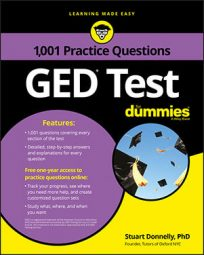When you work with fractions on the GED Math test, you'll often have to reduce them to their lowest common denominator to find the correct answer. The following practice questions will test your reducing skills.

## Practice Questions

1. Four hundred eighty yards is what fraction of a mile? (1 mile = 1,760 yards)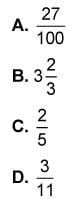Tim measures the lengths of four cats' tails and records his results in the following table.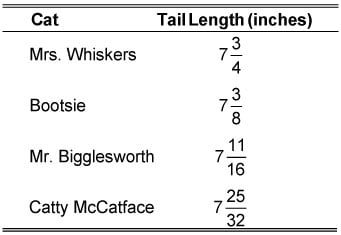2. Which cat has the longest tail? (Note: You may not use a calculator.)

A. Mrs. Whiskers B. Bootsie C. Mr. Bigglesworth D. Catty McCatface

1. The correct answer is D.

Divide the given number of yards by the number of yards in 1 mile: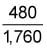Reduce the fraction to its lowest terms. Dividing both the numerator and the denominator by 10 gives you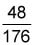Now, dividing both the numerator and the denominator by 8 followed by 2 gives you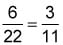Another option is to find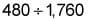Then get a decimal answer and use the calculator's decimal-to-fraction key.

Hence, the correct answer is Choice (D).

2. The correct answer is D.

Because the whole-number part of each cat's tail length equals 7, you can ignore this and focus solely on the fractional part of each length. Hence, you only need to determine which of the following fractions (corresponding to each cat) is the greatest: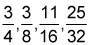The lowest common denominator for all the fractions is 32, so you can rewrite each fraction in terms of this common denominator to get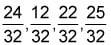Hence, Choice (D) is the correct answer.